﻿ 超空泡航行体自导作用距离需求分析
 舰船科学技术2022, Vol. 44Issue (8): 79-83    DOI: 10.3404/j.issn.1672-7649.2022.08.016PDF

Analysis of necessary homing distance of supercavitating vehicle
XIE Chao, FAN Hua, ZHOU Jing-jun, WAN Ya-min, SONG Shu-long
The 705 Research Institute of CSSC, Xi′an 710077, China
Abstract: In order to carry out the research of homing supercavitating vehicle, it is very essential to evaluate the necessary homing distance. With establishing the supercavitating vehicle dynamic model, this paper calculates the maximum maneuvering angular velocity to be $6°/\mathrm{s}$ based on the control strategy of active bank-to-turn, studies the necessary shortest homing distance under the various situations, and analyzes the initial course of navigation, the initial distance between vehicle and target, etc. The simulation results show that the necessary homing distance of supercavitating vehicle under weak maneuvering conditions is all above 1km, and the current technology cannot meet its homing requirements. Therefore, the supercavitating vehicle weapon represented by the supercavitating torpedo should still attack by direct route in the next period of time.
Key words: supercavitating vehicle     homing distance     active banking strategy
0 引　言

1 超空泡航行体最大机动能力探究 1.1 动力学模型

 $\left\{ \begin{gathered} \dot v = {a_{11}}{B_1} ，\hfill \\ \dot \alpha = {{[( - {a_{11}}\alpha + {a_{12}}){B_1} + {a_{13}}{B_2} + {a_{14}}{B_6}]} \mathord{\left/ {\vphantom {{[( - {a_{11}}\alpha + {a_{12}}){B_1} + {a_{13}}{B_2} + {a_{14}}{B_6}]} v}} \right. } v}，\hfill \\ \dot \beta = {{[( - {a_{11}}\beta - {a_{15}}){B_1} - {a_{13}}{B_3} + {a_{14}}{B_5}]} \mathord{\left/ {\vphantom {{[( - {a_{11}}\beta - {a_{15}}){B_1} - {a_{13}}{B_3} + {a_{14}}{B_5}]} v}} \right. } v}，\hfill \\ {\omega _x} = {{({B_4} - {a_{19}}{B_2} + {a_{20}}{B_3} - {a_{21}}{B_5} - {a_{22}}{B_6})} \mathord{\left/ {\vphantom {{({B_4} - {a_{19}}{B_2} + {a_{20}}{B_3} - {a_{21}}{B_5} - {a_{22}}{B_6})} {{a_{23}}}}} \right. } {{a_{23}}}}，\hfill \\ {\omega _y} = {a_{16}}{B_1} + {a_{14}}{B_3} - {a_{17}}{B_5}，\hfill \\ {\omega _z} = - {a_{18}}{B_1} - {a_{14}}{B_2} + {a_{17}}{B_6}。\hfill \\ \end{gathered} \right.$ (1)

 $\begin{gathered} {B_1} = {f_1}(v,\alpha ,\beta ,{\delta _e},{\delta _r},{\delta _n},\theta ,{\omega _x},{\omega _y},{\omega _z},T)，\hfill \\ {B_2} = {f_2}(v,\alpha ,\beta ,{\delta _e},{\delta _n},\theta ,\psi ,\varphi ,{\omega _x},{\omega _y},{\omega _z}) ，\hfill \\ {B_3} = {f_3}(v,\alpha ,\beta ,{\delta _r},\theta ,\psi ,\varphi ,{\omega _x},{\omega _y},{\omega _z}) ，\hfill \\ {B_4} = {f_4}(v,\alpha ,\beta ,{\delta _b},\theta ,\psi ,\varphi ,{\omega _x},{\omega _y},{\omega _z},{M_{ox}}) ，\hfill \\ {B_5} = {f_5}(v,\alpha ,\beta ,{\delta _b},\theta ,\psi ,\varphi ,{\omega _x},{\omega _y},{\omega _z})，\hfill \\ {B_6} = {f_6}(v,\alpha ,\beta ,{\delta _e},{\delta _n},\theta ,\psi ,\varphi ,{\omega _x},{\omega _y},{\omega _z})。\hfill \\ \end{gathered}$ (2)

1.2 最大机动能力仿真图 1 典型机动弹道曲线 Fig. 1 Trajectory curve of typical maneuver表 1 不同横滚角对应的旋回角速度及最大深度 Tab.1 The angular velocity and maximum depth corresponding to different roll angle

2 需用最短自导作用距离探究 2.1 导引方式

 $1 < p < \frac{2}{\sqrt{1+3{\mathrm{sin}}^{2}\eta }}。$ (3)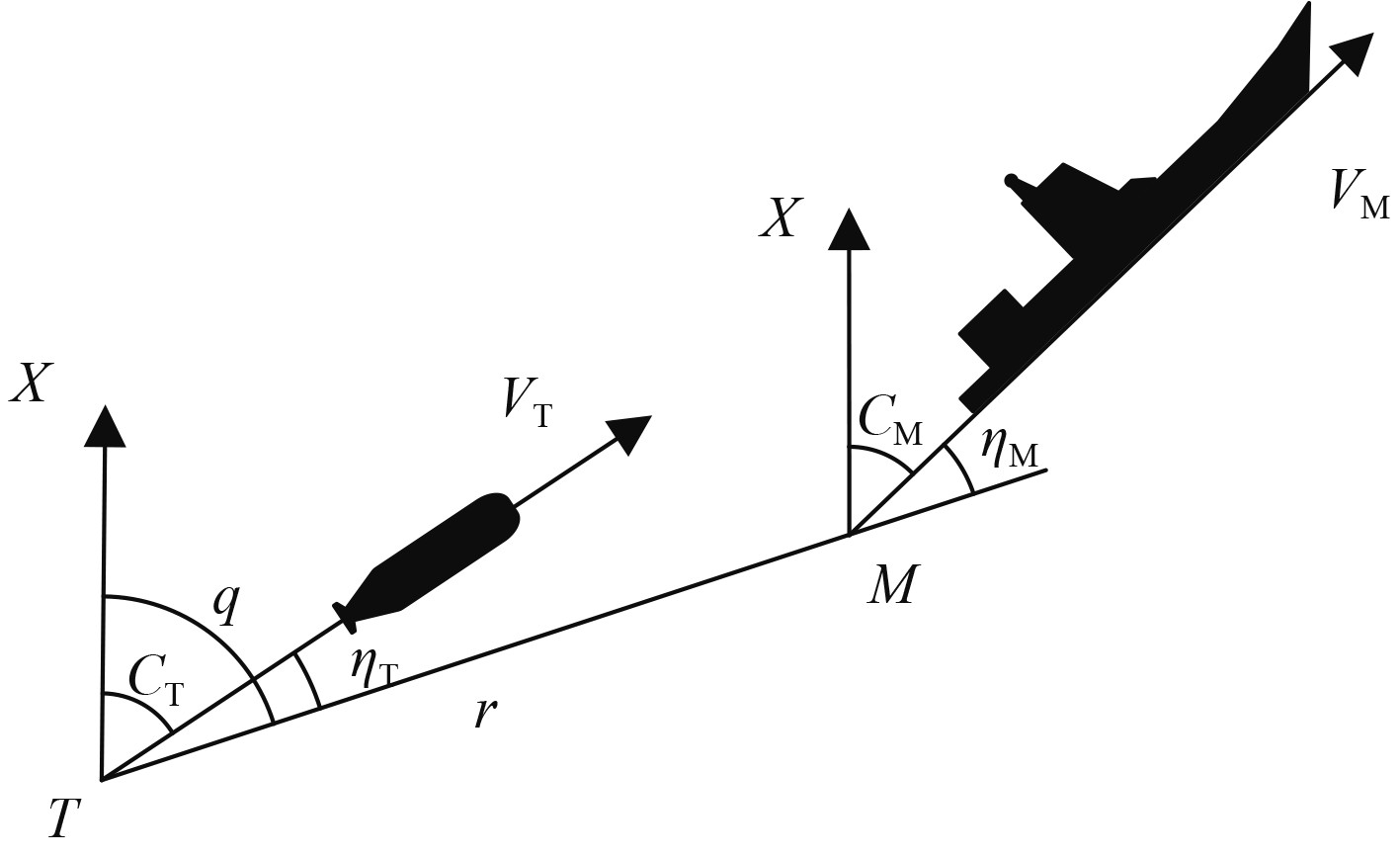图 2 航行体与目标相对运动图 Fig. 2 The relative motion between vehicle and target

 $\left\{\begin{array}{l}\dot{r}={v}_{M}\mathrm{cos}{\eta }_{M}-{v}_{T}\mathrm{sin}{\eta }_{T}，\\ \dot{q}=\dfrac{{v}_{T}\mathrm{sin}{\eta }_{T}-{v}_{M}\mathrm{sin}{\eta }_{M}}{r}，\\ {\eta }_{T}=q-{C}_{T}，\\ {\eta }_{M}=q-{C}_{M}。\end{array}\right.$ (4)

 $\dot{{C}_{T}}=a\cdot \dot{q}，$ (5)

 $\ddot{q}=-\frac{2\dot{r}+a{v}_{T}\mathrm{cos}{\eta }_{T}}{r}\dot{q}+\frac{{v}_{M}\omega }{r}\mathrm{cos}{\eta }_{M}。$ (6)

 $\ddot{q}=-{k}_{1}\dot{q}+{k}_{2} 。$ (7)

 $a > \dfrac{2\left|\dfrac{{\rm{d}}r}{{\rm{d}}t}\right|}{v\cdot \mathrm{cos}{\eta }_{T}} 。$ (8)

 $a=\dfrac{2\left|\dfrac{{\rm{d}}r}{{\rm{d}}t}\right|}{v\cdot \mathrm{cos}{\eta }_{T}}+1。$ (9)
2.2 航行体初始航行段

 ${C}_{T0}={q}_{0}，$ (10)

 ${C}_{T0}={q}_{0}+\mathrm{asin}\frac{\mathrm{sin}\left[{q}_{0}-{C}_{M}-sign({q}_{0}-{C}_{M})\right]}{p} 。$ (11)
2.3 目标机动规避方式

 $\left\{\begin{array}{l} {C}_{M}={C}_{T0}-\theta，-\text{π} \leqslant \alpha \leqslant -\dfrac{\text{π} }{2}，\\ {C}_{M}={C}_{T0}-\text{π} +\theta ，-\dfrac{\text{π} }{2} < \alpha < 0，\\ {C}_{M}={C}_{T0}-\text{π} -\theta ，0\leqslant \alpha < \dfrac{\text{π} }{2}，\\ {C}_{M}={C}_{T0}+\theta ，\dfrac{\text{π} }{2}\leqslant \alpha < \text{π}。\end{array}\right.$ (12)
3 仿真实验表 2 参数设定 Tab.2 Parameter setting
3.1 初始段航向对需用自导距离的影响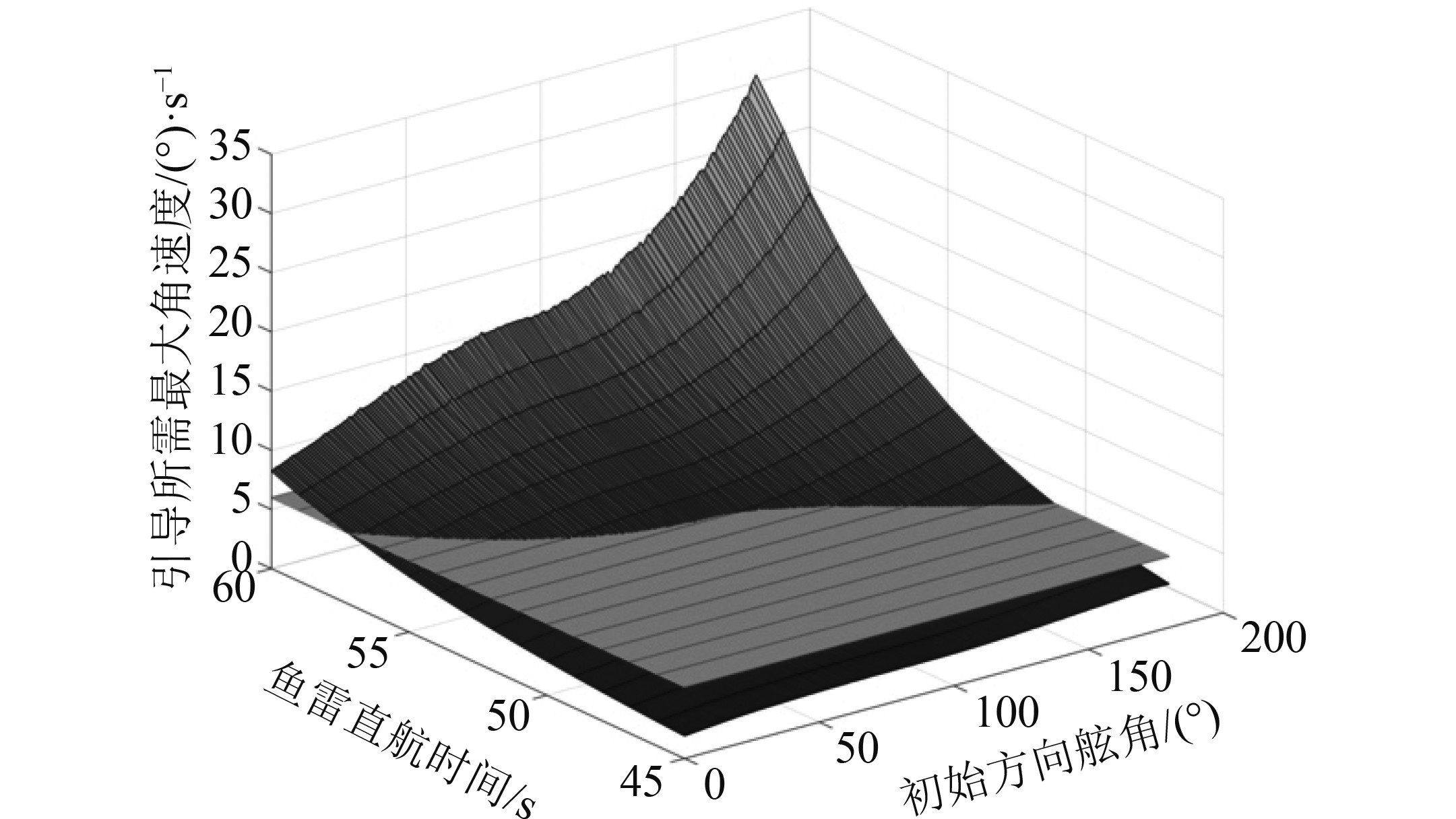图 3 提前角方式 Fig. 3 Ahead position method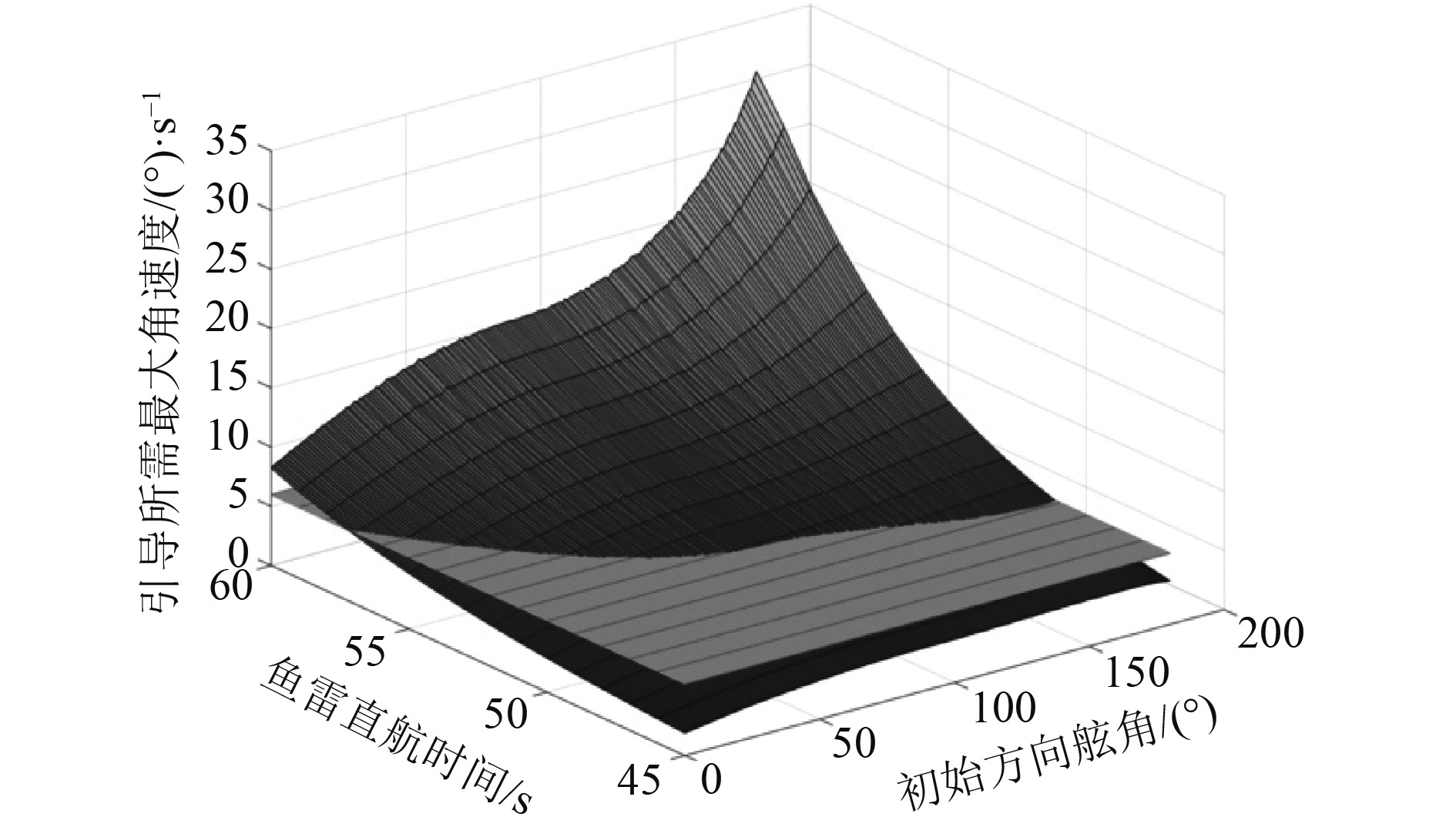图 4 现在位方式 Fig. 4 Current position method

“提前角”方式要求的最短自导作用距离为1771.6 m，对应态势为初始方向舷角30°，航行体直航时间54 s。

“现在位”方式要求的最短自导作用距离为1987.6 m，对应态势为初始方向舷角77°，航行体直航时间50 s。

“提前角”方式要求的自导作用距离比“现在位”的更短，这是因为前者发射方式对目标落点进行了预判，一定程度上减小了航行体在导引过程中需要旋回的角度。

3.2 逃离角度对需用自导距离的影响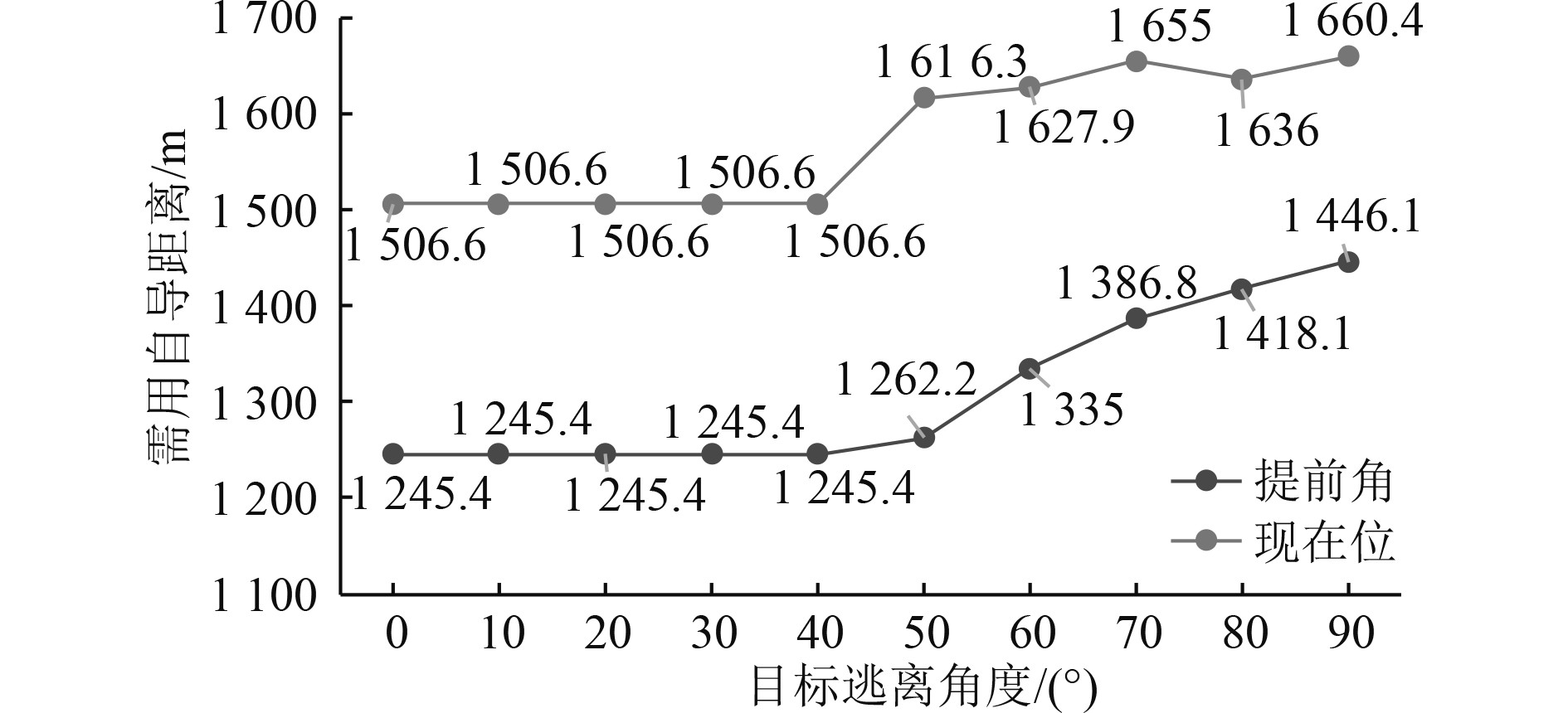图 5 需用自导距离随逃离角度变化图 Fig. 5 Plot of necessary homing distance with escape angle
3.3 初始距离对需用自导距离的影响图 6 需用自导距离随初始距离变化图 Fig. 6 Plot of necessary homing distance with initial distance
3.4 目标机动反应时间对需用自导距离的影响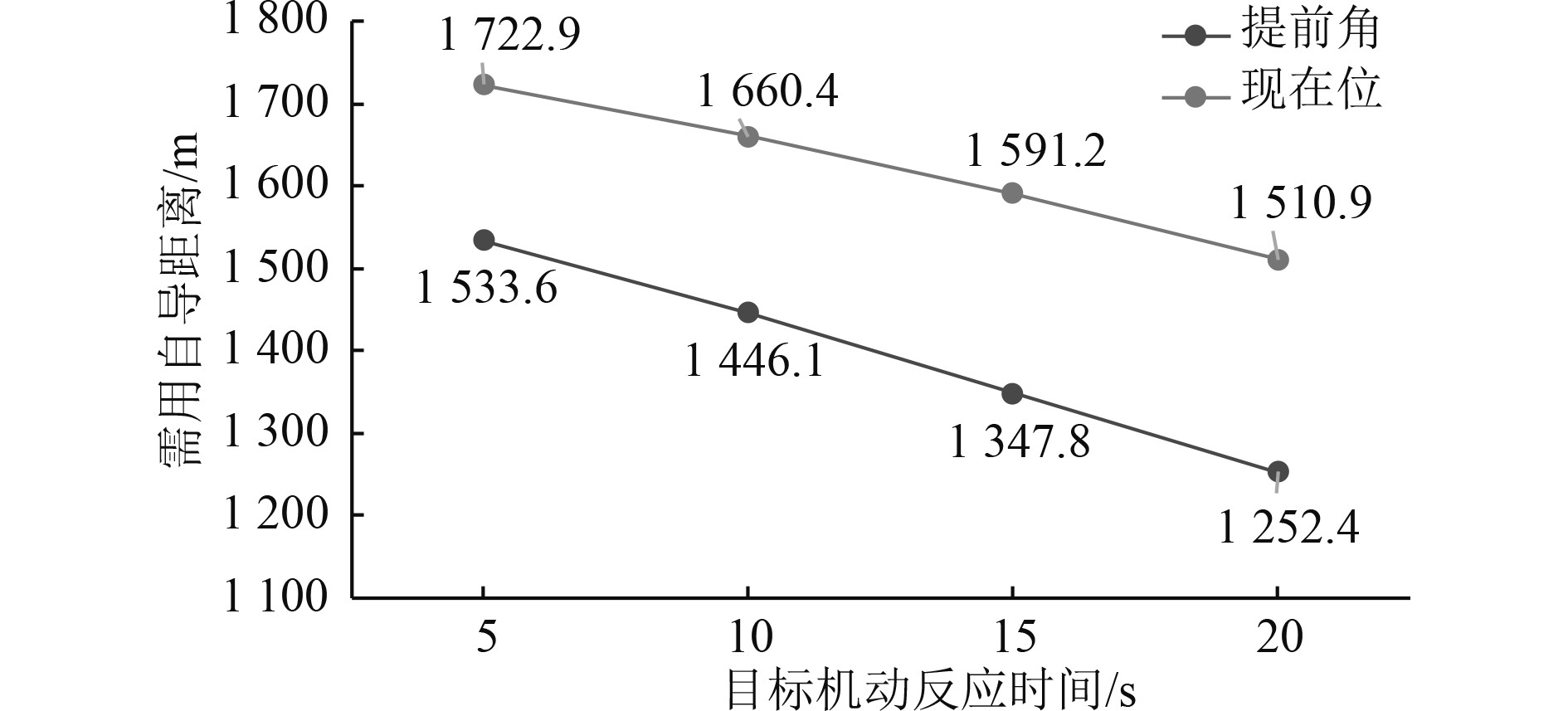图 7 需用自导距离随目标机动反应时间变化图 Fig. 7 Plot of necessary homing distance with target response time to maneuver
3.5 所有态势下的需用自导距离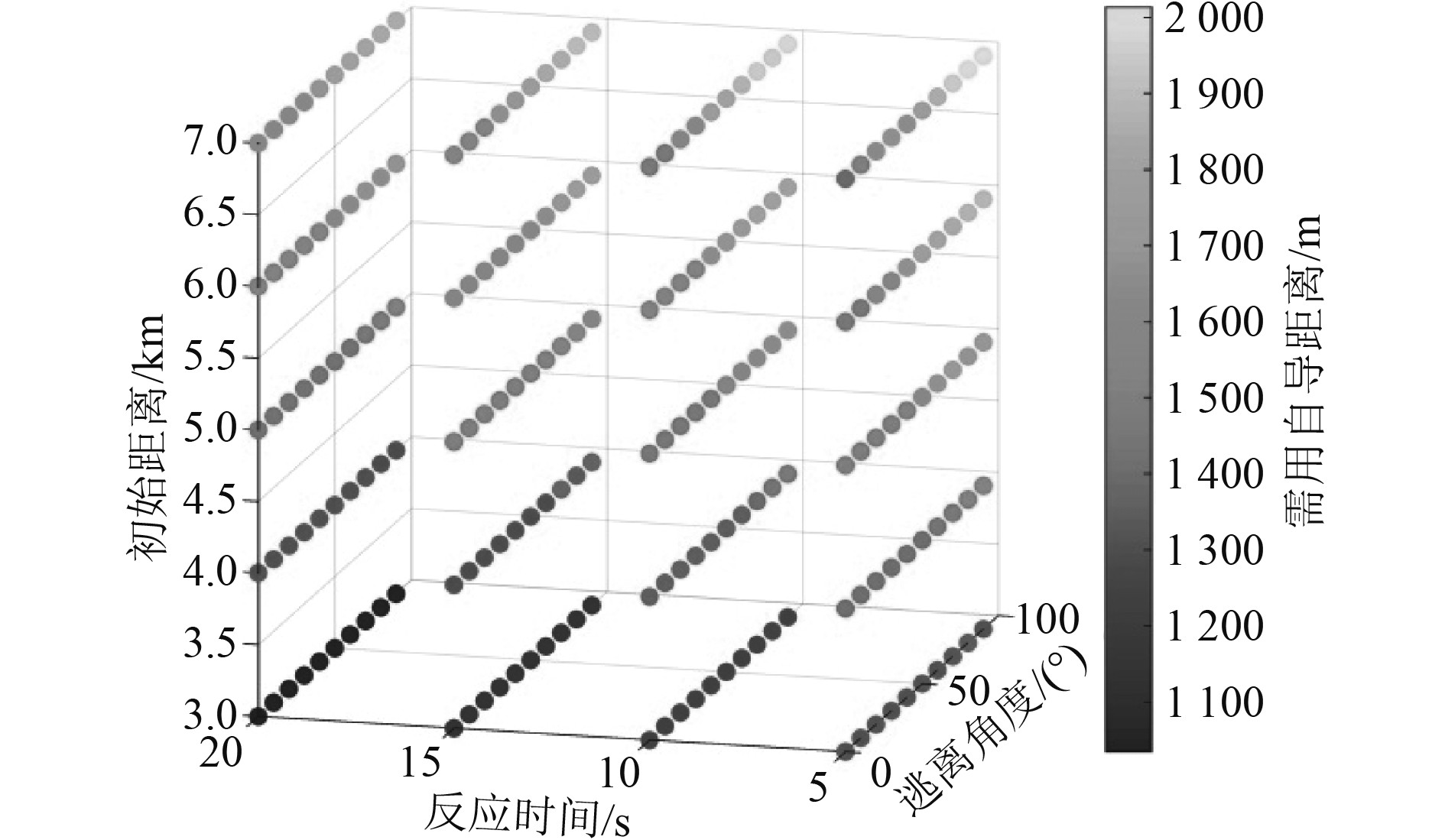图 8 “现在位”攻击的多态势需用自导距离 Fig. 8 Necessary homing distance in various situations through current position method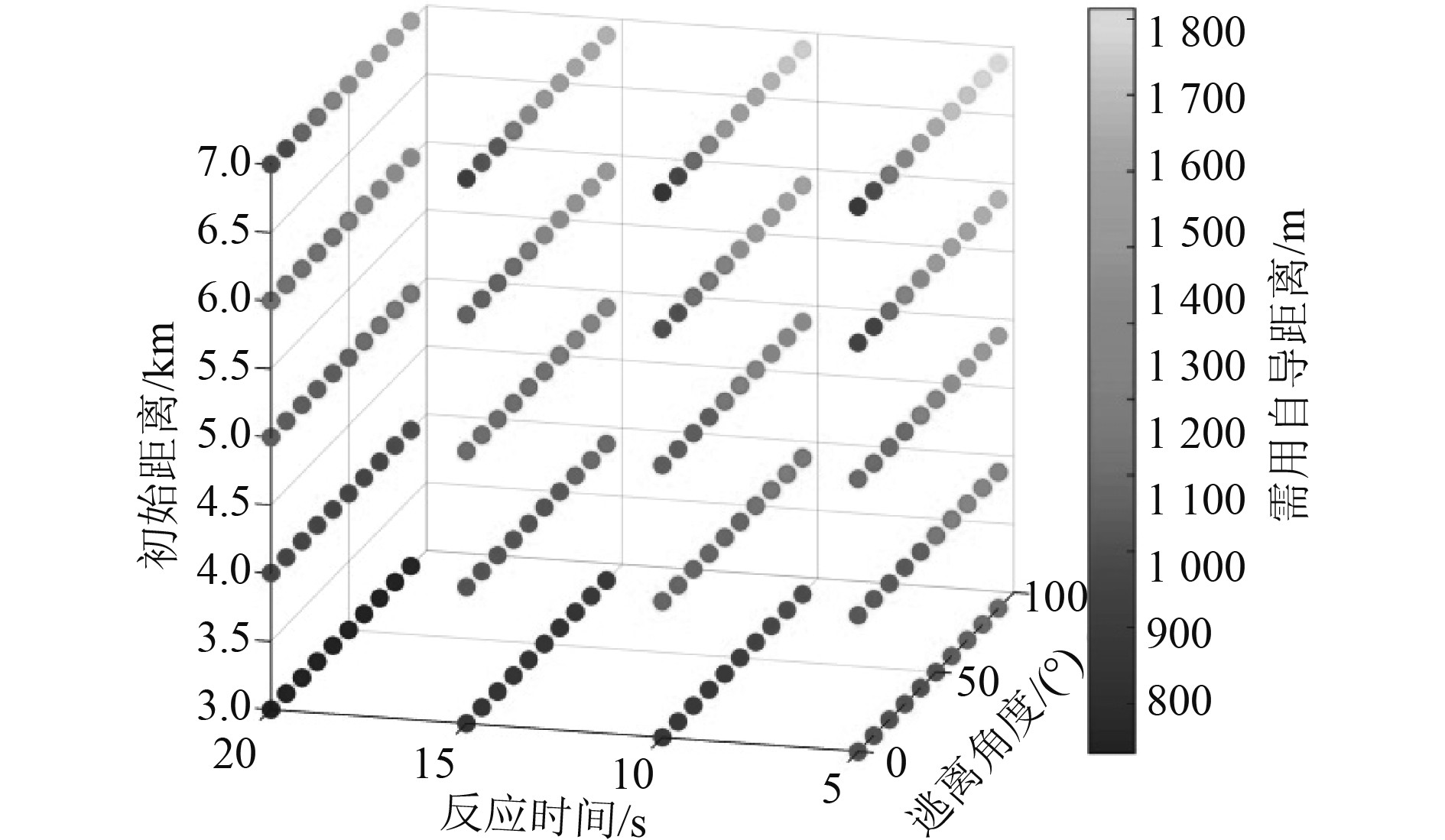图 9 “提前角”攻击的多态势需用自导距离 Fig. 9 Necessary homing distance in various situations through ahead position method

4 结　语

  罗凯, 李代金, 黄闯. 超空泡航行技术的理论基础[M]. 北京: 科学出版社, 2016.  张宇文, 袁绪龙, 邓飞. 超空泡航行体流体动力学[M]. 北京: 国防工业出版社, 2014.  陈颜辉. 水面舰艇综合防御鱼雷行动与技术发展[C]//中国兵工学会、重庆市科学技术协会. OSEC首届兵器工程大会论文集中国兵工学会、重庆市科学技术协会: 兵器装备工程学报编辑部, 2017: 3.  蒋运华, 安伟光, 安海. 超空泡运动体水平面运动弹道特性研究[J]. 弹箭与制导学报, 2012, 32(6): 117-120+124. DOI:10.3969/j.issn.1673-9728.2012.06.035  李代金, 罗凯, 党建军, 等. 超空泡水下航行体空间运动建模与弹道仿真[J]. 兵工学报, 2012, 33(8): 956-961.  罗凯, 党建军, 王育才, 等. 超高速水下航行体纵平面运动特性分析[J]. 鱼雷技术, 2007(5): 37-40.  白涛, 蒋运华, 韩云涛. 基于水洞实验的超空泡形态的动态估计研究[J]. 工程力学, 2017, 34(11): 249-256. DOI:10.6052/j.issn.1000-4750.2016.07.0563  王威, 王聪, 宋武超, 等. 侧滑角对超空泡航行体转弯运动沾湿区域的影响[J]. 振动与冲击, 2020, 39(12): 135-141.  曹立飞, 曹红松, 刘鹏飞, 等. 固定鸭舵二维修正弹比例导引律参数优化[J]. 航空学报, 2021, 42(6): 604-614.  宋书龙, 吕瑞, 周景军, 等. 基于主动倾斜转弯的超空泡航行体机动控制方法[J]. 水下无人系统学报, 2019, 27(6): 607-613.  李洋, 刘明雍, 张小件. 基于自适应RBF神经网络的超空泡航行体反演控制[J]. 自动化学报, 2020, 46(4): 734-743.  陈颜辉. 水面舰艇综合防御鱼雷决策关键技术[J]. 火力与指挥控制, 2019, 44(6): 102-105. DOI:10.3969/j.issn.1002-0640.2019.06.020  邓新文, 朱文振, 谢勇. 潜艇转向旋回防御主动声自导鱼雷的战术意义与作战运用[J]. 水下无人系统学报, 2019, 27(1): 65-70.  王光辉, 宋艳波, 谢宇鹏, 等. 基于突防概率的寻的雷达最优自导距离模型[J]. 弹箭与制导学报, 2010, 30(4): 207-210. DOI:10.3969/j.issn.1673-9728.2010.04.059  陈颜辉, 黄文斌, 孙振新. 最优规避鱼雷策略与最小可规避预警距离[J]. 海军工程大学学报, 2014, 26(2): 76-79+84.